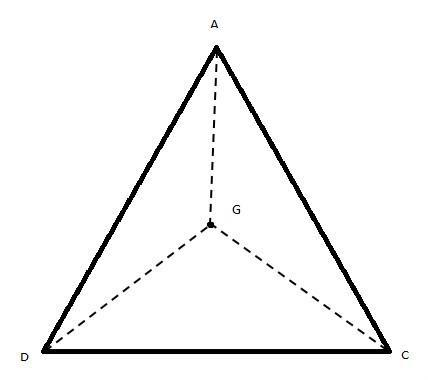QUESTION

# Find the third vertex of a triangle if two of its vertices are at $\left( { - 2,4} \right){\text{ and }}\left( {7, - 1} \right)$ and centroid at $\left( {3,2} \right)$.

Hint: First of all, consider the unknown point as a variable. Then find the centroid of the triangle by using the centroid formula. As we have all points except the third vertex of the triangle, by equating them we will get the coordinates of the third vertex of the triangle. So, use this method to reach the solution of the given problem.

Complete step-by-step solution -
Let the given points are $A{\text{ }}\left( {{x_1},{y_1}} \right) = \left( { - 2,4} \right)$ and $B{\text{ }}\left( {{x_2},{y_2}} \right) = \left( {7, - 1} \right)$
And the centroid is $G{\text{ }}\left( {x,y} \right) = \left( {3,2} \right)$
Consider the required point as $C{\text{ }}\left( {{x_3},{y_3}} \right)$
The diagram of the triangle with centroid is given below:We know that the centroid of a triangle with vertices ${\text{A }}\left( {{x_1},{y_1}} \right)$, ${\text{B }}\left( {{x_2},{y_2}} \right)$ and ${\text{C }}\left( {{x_3},{y_3}} \right)$ is given by the formula ${\text{G }}\left( {x,y} \right) = \left( {\dfrac{{{x_1} + {x_2} + {x_3}}}{3},\dfrac{{{y_1} + {y_1} + {y_1}}}{3}} \right)$.
$\Rightarrow \left( {3,2} \right) = \left( {\dfrac{{ - 2 + 7 + {x_3}}}{3},\dfrac{{4 + \left( { - 1} \right) + {y_3}}}{3}} \right)$
Equating the terms of x-coordinate, we get
$\Rightarrow 3 = \dfrac{{ - 2 + 7 + {x_3}}}{3} \\ \Rightarrow - 2 + 7 + {x_3} = 3 \times 3 \\ \Rightarrow 5 + {x_3} = 9 \\ \therefore {x_3} = 9 - 5 = 4 \\$
Equating the terms of y-coordinate, we get
$\Rightarrow 2 = \dfrac{{4 + \left( { - 1} \right) + {y_3}}}{3} \\ \Rightarrow 4 + \left( { - 1} \right) + {y_3} = 2 \times 3 \\ \Rightarrow 3 + {y_3} = 6 \\ \therefore {y_3} = 9 - 3 = 3 \\$
Thus, the third vertex of the triangle is $C{\text{ }}\left( {{x_3},{y_3}} \right) = \left( {4,3} \right)$.

Note: The centroid of a triangle with vertices ${\text{A }}\left( {{x_1},{y_1}} \right)$, ${\text{B }}\left( {{x_2},{y_2}} \right)$ and ${\text{C }}\left( {{x_3},{y_3}} \right)$ is given by the formula${\text{G }}\left( {x,y} \right) = \left( {\dfrac{{{x_1} + {x_2} + {x_3}}}{3},\dfrac{{{y_1} + {y_1} + {y_1}}}{3}} \right)$. Or else, we can the third vertex directly by using the formula ${\text{C }} = 3{\text{G}} - \left( {{\text{A}} + {\text{B}}} \right)$.